# Numerical Simulation of Energy Systems to Control Environment Microclimate

Numerical Simulation of Energy Systems to Control Environment Microclimate

Angela GencoAnnarita Viggiano Lucio Viscido Giuseppe Sellitto Vinicio Magi

School of Engineering – University of Basilicata, via dell’Ateneo Lucano 10, Potenza 85100, Italy

CRF/WCM R&I – Campus Manufacturing, Str. Prov. Casamassima, Valenzano 70010, Italy

CRF/WCM R&I – Campus Manufacturing, via ex-Aeroporto, Pomigliano D’Arco 80038, Italy

Corresponding Author Email:
angela.genco@unibas.it
Page:
S545-S552
|
DOI:
https://doi.org/10.18280/ijht.34S249
|
Accepted:
|
Published:
31 October 2016
| Citation

OPEN ACCESS

Abstract:

The aim of the present work is the development of a predictive mathematical model for the analysis and optimization of energy systems used to control the environment microclimate in industrial plants. This model provides not only the evaluation of the optimal configuration on the basis of different process parameters in the existing environments, but also the analysis and the prediction of the energy consumption of a plant during the design phase. The model describes the thermodynamics of conditioning processes and allows the evaluation of the influence of design variables and of hourly averaged weather conditions on energy consumption. The model is developed both in TRNSYS-17 and C ++ programming language such that it can also be used under MATLAB computing environment. The results obtained with the two models are compared under different climatic conditions in terms of heating/cooling and humidification/dehumidification energy consumption, thus assessing the accuracy of both models. The results obtained by using different set-point conditions under different climatic zones are also presented.

Keywords:

Dynamic simulation, Air conditioning, Microclimate, Energy efficiency.

1. Introduction

It is well known that the air quality of the environment where people live or work affects the well-being of the occupants, as well as, in the industrial sector, the microclimatic conditions may affect in some cases the performance of the production process. Specifically, the microclimatic control is mandatory, for instance, in the food industry, in some phases of the productive chain of the automotive sector, in the textile sector, in hospital buildings and, more in general, in all accommodation facilities. All the aforementioned cases require the attainment of specific conditions for temperature and humidity. On the other hand, the components of the energy systems to control such environments should be properly designed and optimized in order to reduce the energy consumption and pollutant emissions.

As far as building energy systems are concerned, an interesting review of existing models for the simulation of energy plants is given in Ref. . In Ref. , a direct model and an inverse model are coupled together to determine the thermal characteristics of buildings. A methodology that allows to define a series of dwelling type as a function of building, family and environmental characteristics is developed in Ref. . Then, for each dwelling type the monthly energy consumption is estimated. The development of predictive models by using measured and/or forecast weather data is fundamental in order to efficiently control the energy performance of such systems, as shown in Ref. . For example, these models can be used to optimize the smart energy grids and reduce the energy consumption , to manage large scale solar plants for industrial applications  or to analyze energy consumption of buildings under different climatic conditions as given in Ref. .

Usually, these energy systems are composed of several devices in order to achieve the set-point of thermo-hygrometric conditions. Hence, it is required to keep track of the energy behavior of each device. In Ref. , the energy system has been modeled through a modular mathematical approach for industrial plant simulation. Specifically, each device is described by a set of equations based on mechanical and thermodynamic laws. In Ref. , the authors have developed a model in order to simulate solar plants for industrial heat applications. Such a model provides an energy consumption monitoring of the industrial plants and an optimization of the energy performance of the entire system. In Ref.  a micro-CHP plant powered with syngas is used to produce electrical and thermal energy for small agro-industries. In this case, a balance of mass and energy is employed to compute the performance of the system. In Ref. , a thermodynamic model of a solar hybrid photovoltaic-thermal solar system is given. Specifically, the Simulink/MATLAB computing environment is used to model the system components by means of a modular approach.

The aim of this work is the development of a predictive mathematical model for the analysis and optimization of the components of the energy systems employed to control the microclimate of industrial plants. The entire system is simulated by using both the simulation software TRNSYS-17 [11-14] and an in-house code written in C++ programming language so that it can also be used under MATLAB computing environment [15-16].

Among the features of the TRNSYS software, it is worth mentioning i) the availability of the source code and of a good technical documentation, ii) the modular design which allows to represent the building and the plant through components (called type) and iii) the ability to connect to other software such as TRNBUILD and Excel. As regards MATLAB, it is well known that this computing environment allows to implement computational models by using either available mathematical libraries or by writing new libraries from scratch.

In the present work, a customized simulation model has been developed to assess the energy performance of the entire energy system in terms of heating/cooling and humidification/dehumidification energy consumption. The simulations are performed under dynamic conditions by using a 1-hour simulation time step and measured weather data. The model includes both the air conditioning plant and the conditioned environment. As far as the air conditioning plant is concerned, temperature and relative humidity of the air that flows into the conditioned environment must be strictly controlled. As an output, the simulation model provides the energy consumption of the air conditioning plant, the heat transferred between the controlled microclimatic environment and its surrounding and the values of temperature and relative humidity coming from the conditioning process. The model describes the thermodynamics of conditioning process of the environment and allows the evaluation of the influence of design variables and of hourly averaged weather conditions on energy consumption. In this work, the results obtained by using both a C++ code and TRNSYS-17 are compared in terms of energy consumption, temperature and relative humidity coming from the conditioning process to validate both models.

In order to evaluate the energy consumption of such energy systems under different external climatic conditions, simulations are carried out by considering three different climatic zones of Italy and three different set-point conditions of temperature and relative humidity. Specifically, three different cities of northern, central and southern Italy, i.e. Turin, Rome and Potenza, are considered. The three set-point conditions considered are as follows: i) temperature range 10°C to 15°C and relative humidity 30% to 40%, ii) temperature 20°C to 25°C and relative humidity 40% to 50% and iii) temperature 35°C to 40°C and relative humidity 60% to 65%. These three different conditions are chosen to account for some of the human/industrial activities that require careful management of the indoor microclimate such as food industries, the painting process in industrial plants and greenhouse farming. The results of the entire set of different simulations are compared and discussed.

This work is organized as follows: first the simulation model is described, then the results are discussed in terms of model validation and sensitivity to climatic and set-point conditions, and, finally, conclusions are summarized.

2. The Model

In this section, a detailed description of the model to simulate the air conditioning system is given. Specifically, the air conditioning plant has been analyzed by using the mathematical equations that describe the thermo-hygrometric behavior of the moist air. Such equations are used to set the air temperature in the first step and relative humidity in the second step based on the set-point conditions.

First of all, the model reads the average hourly weather data, in terms of air temperature and relative humidity. Then, the temperature of the air mass flow rate is adjusted to get a temperature within the set-point range, whereas the relative humidity is set to the value corresponding to the temperature reached by the air. Based on the temperature bandwidth Tmin,setpoint ÷ Tmax,setpoint , if the initial air temperature is lower than Tmin,setpoint the heating unit heats the air to reach Tmin,setpoint; conversely, if the input temperature is higher than Tmax,setpoint the cooling unit cools the air to reach Tmax,setpoint . In order to achieve the final temperature within the range Tmin,setpoint ÷ Tmax,setpoint , it is necessary to provide or remove thermal energy by the air.

In the heating process, thermal energy is provided to the moist air in order to increase its temperature. The thermal energy is computed according to:

$\dot{Q}_{\text {heat}}=\dot{m}_{\text {air}} \cdot\left(h_{\text {out}}-h_{\text {in}}\right) \quad[\mathrm{kJ} / {\mathrm{h}}]$(1)

where:

$\dot{m}_{a i r}$  is the dry air mass flow rate to be conditioned [kg/h];

$h_{o u t}$  is the final enthalpy of dry air and water vapor mixture [kJ/kg];

$h_{i n}$  is the initial enthalpy of dry air and water vapor mixture [kJ/kg].

On the other hand, the cooling process consists of removing thermal energy from the moist air to decrease its temperature. The thermal energy for this process is computed according to:

$\dot{Q}_{c o o l}=\dot{m}_{a i r} \cdot\left(h_{i n}-h_{o u t}\right) \quad[\mathrm{kJ} / {h}]$(2)

where:

$\dot{m}_{a i r}$  is the dry air mass flow rate to be conditioned [kg/h];

$h_{o u t}$  is the final enthalpy of dry air and water vapor mixture [kJ/kg];

$h_{i n}$  is the initial enthalpy of dry air and water vapor mixture [kJ/kg].

The enthalpy of dry air and water vapor mixture is computed according to:

$h=h_{a}+h_{v} \quad[\mathrm{kJ} / {\mathrm{kg}}]$(3)

and

$h_{a}=c_{p a} \cdot T \quad[\mathrm{kJ} /{\mathrm{kg}}]$(4)

$h_{v}=x \cdot r+x \cdot c_{p v} \cdot T \quad[\mathrm{kJ} / {\mathrm{kg}}]$(5)

where:

$h_{a}$  is the enthalpy of dry air [kJ/kg];

$h_{v}$  is the enthalpy of water vapor [kJ/kg];

$c_{p a}$  is the specific heat of dry air at constant pressure [kJ/(kg K)];

$c_{p v}$  is the specific heat of water at constant pressure [kJ/(kg K)];

$\chi$  is the humidity ratio of the mixture [kgH2O/kg dry air];

$r$  is the latent heat of vaporization [kJ/kg].

The latent heat of vaporization r is computed by using the following mathematical expression:

$r=2500.8-2.36 \cdot T+0.0016 \cdot T^{2}-0.00006 \cdot T^{3}[\mathrm{kJ} / \mathrm{kg}]$(6)

where T is the temperature in degree Celsius.

During the heating process, an increase in the air temperature yields to a decrease in its relative humidity. This is because the amount of the water vapor mass at saturation condition increases, whereas the actual water vapor mass within the moist air remains the same. Therefore, at the end of the heating process, if the air relative humidity is lower than the minimum set-point value, the model allows to increase such a humidity to reach the minimum set-point value.

On the other hand, during the cooling process the air temperature decreases with an increase of its relative humidity. If the temperature overcomes the dew-point value, a part of the water vapor within the moist air condenses. Therefore, at the end of the cooling process, if the relative humidity is higher than the maximum set-point value, the model allows to decrease such a humidity to reach the maximum set-point value.

The dew-point temperature is calculated according to Eq. (7), which is valid within the temperature range 0÷93°C :

$T_{r}=c_{14}+c_{15} \cdot \alpha+c_{16} \cdot \alpha^{2}+c_{17} \cdot \alpha^{3}+c_{18} \cdot\left(p_{v}\right)^{0.1984}\left[^{\circ} \mathrm{C}\right]$(7)

where:

$p_{v}$  is the partial pressure of water vapor [kPa];

$\alpha=\log \left(p_{v}\right)$ ;

$c_{14}=6.54$ ;

$c_{15}=14.526$ ;

$c_{16}=0.7389$ ;

$c_{17}=0.09486$ ;

$c_{18}=0.4569$ .

The second unit of the air conditioning system modifies the relative humidity of the air mass flow rate and returns air temperature and humidity values within the respective set-point ranges. Given the relative humidity set-point range U.R.min,setpoint ÷ U.R.max,setpoint , if the initial relative humidity is lower than U.R.min,setpoint the unit humidifies the air to reach U.R.min,setpoint, whereas, if the input relative humidity is higher than U.R.max,setpoint the unit dehumidifies the air to reach U.R.max,setpoint .

In order to achieve the relative humidity within the set-point range, it is necessary to provide/subtract energy to/from the air mass flow rate. The humidification process provides energy to the air in order to increase its humidity. The energy required for this process is computed according to:

$\dot{Q}_{\text {humidification}}=\dot{m}_{\text {air}} \cdot\left(h_{\text {out}}-h_{\text {in}}\right)[\mathrm{kJ} / \mathrm{h}]$(8)

whereas the thermal energy for the air dehumidification is computed according to:

$\dot{Q}_{\text {dehumidification}}=\dot{m}_{a i r}\left(h_{i n}-h_{\text {out}}\right) \quad[\mathrm{kJ} / \mathrm{h}]$(9)

where:

$\dot{m}_{a i r}$  is the moist air mass flow rate to be conditioned [kg/h];

$h_{o u t}$  is the final enthalpy of dry air and water vapor mixture [kJ/kg];

$h_{i n}$  is the initial enthalpy of dry air and water vapor mixture [kJ/kg].

It is worth outlining that Eqns. (1) and (8) and Eqns. (2) and (9) are based on different initial air conditions. Specifically, in the heating/cooling process the air temperature and relative humidity are referred to external conditions, whereas in the humidification/dehumidification process the initial air temperature and relative humidity are those at the end of the heating/cooling process.

At the end of the cooling and dehumidification processes, the moist air is characterized by its relative humidity within the set-point range whereas the temperature could not. Therefore, a possible reheating of the mixture should be taken into consideration. This reheat is a process that occurs at constant absolute humidity. The reheat energy is computed according to:

$\dot{Q}_{\text {reheat}}=\dot{m}_{\text {air}}\left(h_{\text {reheat}}-h_{\text {out}}\right) \quad[\mathrm{kJ} / {\mathrm{h}}]$(10)

where hout [kJ/kg] is the enthalpy at the end of the dehumidification process and hreheat is the enthalpy at the end of the reheat process.

The air mass flow rate is provided by a variable speed fan, which is placed downstream of the air conditioning system, in order to draw the air flow from the outside thru the air conditioning devices to the conditioned environment. The fan increases the air pressure to balance the distributed and concentrated load losses due to distribution channels, change of sections, fittings etc. The air mass flow rate of the fan is computed using:

$\dot{m}=\dot{m}_{\text {rated}} \cdot \gamma[\mathrm{kg} / {\mathrm{h}}]$(11)

where:

$\dot{m}_{\text {rated}}$   is the fan nominal air mass flow rate [kg/h];

$\gamma$   is a control signal within the range [0÷1] that adjusts the amount of air to be transferred.

The power supplied by the fan is evaluated with:

$\dot{P}=\dot{P}_{\text {rated}} \cdot\left(\alpha_{0}+\alpha_{1} \cdot \gamma+\alpha_{2} \cdot \gamma^{2}+\alpha_{3} \cdot \gamma^{3}\right) \quad[\mathrm{kJ} / \mathrm{h}]$(12)

where:

$\dot{P}_{\text {rated}}$  is the nominal power of the fan [kJ/h];

$\alpha_{0}, \alpha_{1}, \alpha_{2}, \alpha_{3}$  are coefficients of the polynomial function which depend on the actual fan mode of operation. In the model a polynomial relationship of the first order is applied, i.e. $\alpha_{0,2,3}=0$ and $\alpha_{1}=1$  .

The environment with controlled micro-climatic conditions is simulated as a room with specified thermal loss features. Hence, inside the conditioned environment, the air may change its thermodynamic properties due to such losses. The thermal losses are evaluated with:

$\dot{Q}=\sum_{\mathrm{i}=1, \ldots, \mathrm{n}}\left(\mathrm{U}_{\mathrm{i}} \cdot \mathrm{A}_{\mathrm{i}} \cdot \Delta \mathrm{T}\right)[\mathrm{W}]$(13)

where:

Ui is the heat transfer coefficient of the i-th wall element of the environment [W/m2 K];

Ai is the area of the i-th wall element of the environment [m2];

$\Delta \mathrm{T}$  is the temperature difference between the conditioned environment and its surrounding [K];

n is the total number of wall elements.

## figure_1.jpg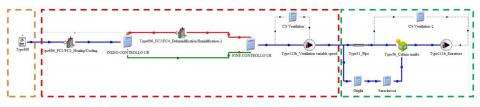Figure 1. Simulation model layout with TRNSYS-17

Finally, the air extraction system takes care of the moist air which leaves the conditioned environment. Such a system consists of a variable speed fan and a set of filters to allow a primary filtering of the air due to the presence of possible polluting particles.

The layout of the model under TRNSYS-17 environment is shown in Figure 1. The model is composed of the following three parts: weather data reader (framed with orange line), the first air conditioning device in order to adjust the air temperature, the second air conditioning device in order to adjust the air relative humidity and the variable speed fan (framed with red line), the distribution system, the controlled micro-climatic environment and the final variable speed fan (framed with green line). Both in TRNSYS-17 and in C++ code, the weather data input file is provided in .EPW format.

3. Results

3.1 Model validation

In order to validate the model and its implementation, simulations of the air conditioning system described in the previous section have been carried out by considering the weather conditions of the city of Potenza in Italy. Specifically, the computations were carried out for a three-day time period of May (average weather data for the years 1951-1970 ) based on the operating conditions given in Table 1. This test case will be referred to as a baseline case in what follows.

The results of the simulations obtained by using the C++ code developed in this work, named AC_Code in what follows, and those obtained by using the implementation in TRNSYS-17 are compared for validation. In order to make a fair comparison, in the AC_Code, the latent heat of vaporization has been set to a constant value, equal to 2,501 kJ/kg , which is the value used in TRNSYS-17.

Figure 2 shows the results in terms of energy consumption for the selected three-day time period. The total energy consumption consists of heating, cooling, humidification, dehumidification and reheat energy contributions. The results obtained by using the two implementations are very close in terms of heating/cooling and humidification/dehumidification consumption, whereas there are some differences in terms of reheat energy consumptions. This is due to a different choice in the set-point conditions operated by TRNSYS-17 code with respect to AC_Code. Figure 2 shows that the most part of energy is required during heating, whereas cooling, humidification and reheat energy requests are less. The dehumidification process contributes to the total energy consumption to a small extent.

Figure 3 shows the hourly energy consumption versus time in the three-day time period of May in terms of heating and cooling energy consumption. Analogously, Figure 4 shows some details about the energy consumption in the same period of time as regards humidification and dehumidification. This time frame has been chosen because it includes all forms of energy consumption i.e. heating/cooling and humidification/ dehumidification. Figures 3 and 4 show that the results obtained with AC_Code and those obtained with TRNSYS-17 are on top of each other.

Table 1. Operating conditions for the model validation

 City Potenza Temperature range 22÷25 °C Relative humidity range 55÷60 % Air mass flow rate 47,000 kg/h Rated fan power 25 kW Motor efficiency 0.9 Motor heat losses fraction 0.1 Simulation time 1÷72 h

## figure_2.png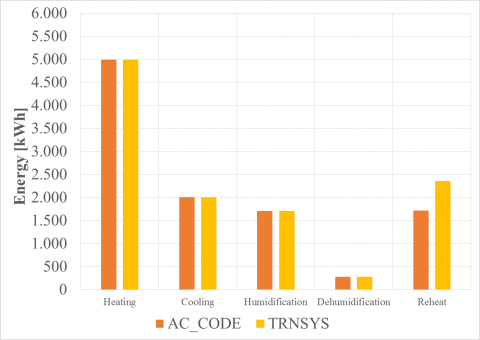Figure 2. Baseline case: energy consumption for the three-day time period of May obtained with AC_Code and TRNSYS-17

3.2 Sensitivity to different climatic conditions

In order to analyze the performance of the air conditioning system under different climatic conditions, three Italian cities, i.e. Turin, Rome and Potenza, have been considered, which are characterized by climatic conditions quite different from each other. The weather conditions during the one-year time period for the three cities are extracted from the database of the Italian Climatic data collection “Gianni De Giorgio” (IGDG) based on a 1951-1970 time period of records, available at the link https://energyplus.net/weather/sources . For instance, Figures 5 and 6 show the climatic conditions of the three cities in January by considering average yearly weather data for the years 1951-1970. Specifically, Figure 5 shows the outside air temperature, while Figure 6 shows the outside air relative humidity in the same time frame. In addition, Figures 7 and 8 show the climatic conditions of the three cities in July by considering average yearly weather data in the period 1951-1970. Figure 7 shows the outside air temperature, whereas Figure 8 shows the outside air relative humidity. In all figures, gray color curves refer to Potenza, blue color curves refer to Rome and green color curves relate to Turin.

## figure_3.png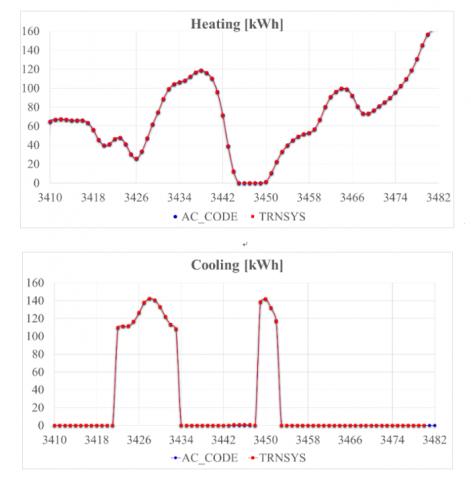Figure 3. Baseline case: heating/cooling energy consumption vs time in the three-day time period of May obtained with AC_Code and TRNSYS-17

## figure_4.png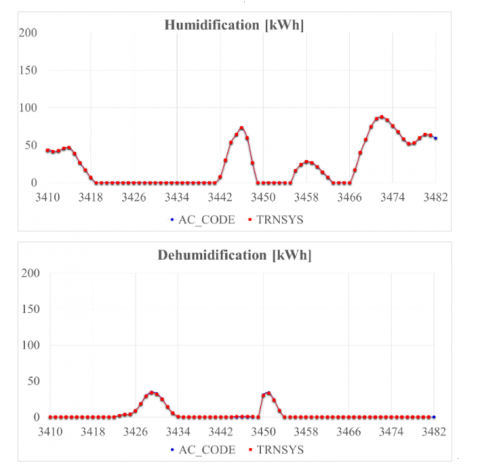Figure 4. Baseline case: humidification/dehumidification energy consumption vs time in the three-day time period of May obtained with AC_Code and TRNSYS-17

## figure_5.png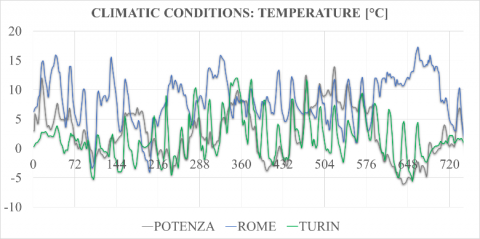Figure 5. January: outside air temperature in Potenza, Rome and Turin

## figure_6.png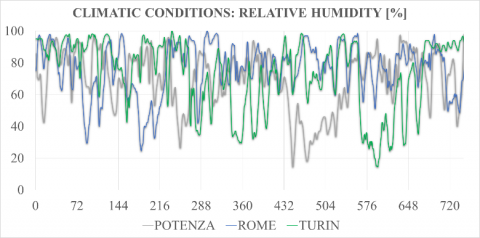Figure 6. January: outside air relative humidity in Potenza, Rome and Turin

## figure_7.png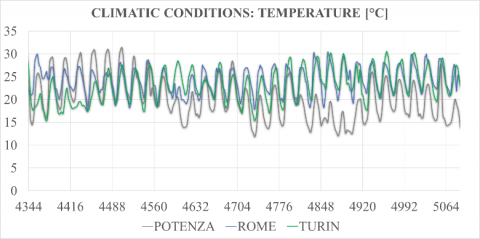Figure 7. July: outside air temperature in Potenza, Rome and Turin

## figure_8.png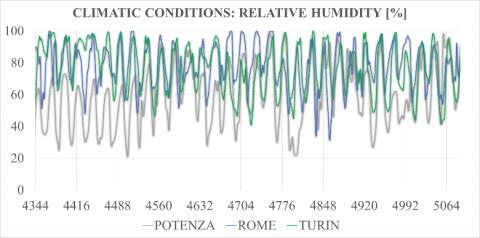Figure 8. July: outside air relative humidity in Potenza, Rome and Turin

The energy requirement has been computed by using the AC_Code under the assumption that the air conditioning system was located in the three cities. Table 2 summarizes the operating conditions for the three simulations.

Table 2. Operating conditions for the sensitivity analysis to climatic conditions

 City Turin, Rome, Potenza Temperature range 22÷25 °C Relative humidity range 55÷60 % Air mass flow rate 47,000 kg/h Rated fan power 25 kW Motor efficiency 0.9 Motor heat losses fraction 0.1 Simulation time 1÷8,760 h

The results are given in Figure 9 and are compared in terms of total energy consumption. Such results are obtained by considering the three different climatic conditions during a one-year time period (average yearly data for the years 1951-1970). The results show that the energy consumption in Turin, which is located in northern Italy, is higher than the energy consumption in Potenza and Rome, which are located in southern and central Italy, respectively.

This is an interesting result even if Turin and Potenza belong to the same climatic zone according to the current Italian legislation .

## figure_9.png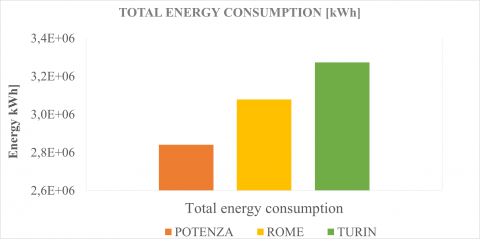Figure 9. Total energy consumption in Potenza, Rome and Turin

Further considerations can be drawn from Figure 10, where the total energy consumption is divided into heating, cooling, humidification, dehumidification, reheat, together with the two fans energy contributions. The orange, yellow and green color columns refer to Potenza, Rome and Turin, respectively. Figure 10 shows that the heating and humidification energy consumption in Potenza is higher than in the two other cities, due to the relative cold winter and mild summer that characterize the climate in Potenza, whereas cooling, dehumidification and reheat contributions are relatively low. This is also confirmed in Figures 11-13, where the different contributions are represented on a percentage basis. Moreover, Figure 10 shows that the humidification energy follows the same trend of the heating consumption, since usually the heating process is coupled with the humidification process. On the other hand, cooling, dehumidification and reheat show the same trend, since cooling is usually followed by dehumidification and, eventually, by reheat. Specifically, reheat process can occur only after the cooling/dehumidification process is completed in order to increase the air temperature and reach the set-point conditions.

Furthermore, Figure 10 shows that, among the three cities, Rome is characterized by the highest demand in terms of cooling, dehumidification and reheat energy. In fact, as also shown in Figure 12, the energy request in Rome is more uniformly distributed in terms of different contributions with respect to Potenza, even if the highest energy consumption is due to the heating process, followed by the cooling process.

On the other hand, the energy request of Turin is between Potenza demand and Rome demand for all the energy contributions, except for the fans energy consumption which is constant for all cities, since it does not depend on the climatic conditions. On a percentage basis, the energy consumption in Turin is divided among the different processes similarly to Potenza, as shown in Figure 13. In fact, the highest energy request is due to the heating and humidification processes.

## figure_10.pngFigure 10. Sensitivity of energy consumption to different climatic conditions

## figure_11.png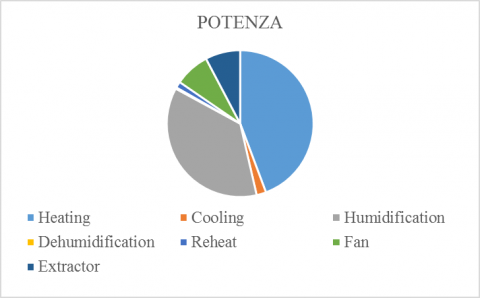Figure 11. Contributions to energy consumption – Potenza

## figure_12.png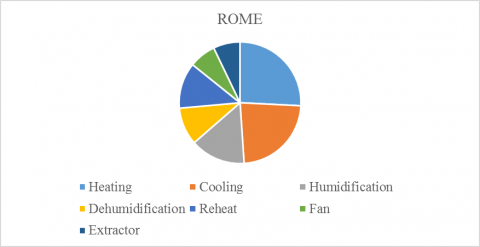Figure 12. Contributions to energy consumption – Rome

## figure_13.png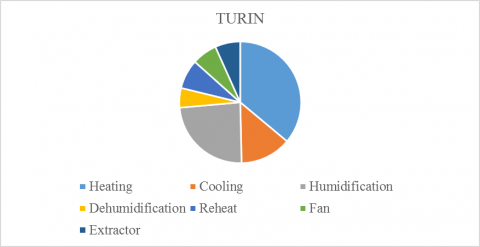Figure 13. Contributions to energy consumption – Turin

3.3 Sensitivity to set-point conditions

In order to analyze the energy consumptions of the air conditioning system under different set-point conditions, three cases have been considered by using the weather data of Potenza. The three different set-point conditions are given in Table 3, together with the entire set of operating conditions. Case A refers to the lowest temperature and relative humidity ranges, Case C accounts for the highest temperature and relative humidity ranges, whereas Case B is intermediate between the other two cases.

Table 3. Operating conditions for the sensitivity analysis to different set-point conditions

 City Potenza Temperature range Case A: 10÷15 °C Case B: 20÷25 °C Case C: 35÷40 °C Relative humidity range Case A: 30÷40 % Case B: 40÷50 % Case C: 60÷65 % Air mass flow rate 47,000 kg/h Rated fan power 25 kW Motor efficiency 0.9 Motor heat losses fraction 0.1 Simulation time 1÷8,760 h

The results of the simulations obtained by using AC_Code are shown in Figure 14. The orange color column is referred to Case A, the yellow color column regards Case B, whereas the green color column is referred to Case C. By increasing/decreasing the set-point temperature range, the energy demand for the heating/cooling process increases. Thus, Case A is characterized by the highest cooling energy request compared to the other two cases, whereas Case C does not require energy for cooling since the outside air temperature is always lower than Tmax, set-point. The humidification energy request follows a trend similar to the heating energy request since in all cases the relative humidity set-point range increases with the temperature range. Besides, the cooling and the dehumidification processes have a similar trend. The reheat energy demand follows the same trend of the dehumidification process, as expected. Again, fans energy consumptions do not depend on the set-point conditions.

## figure_14.png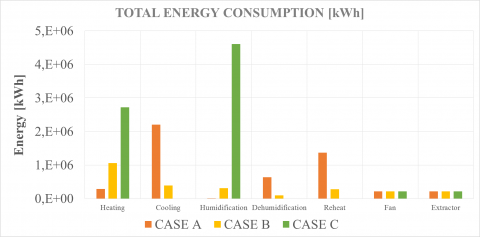Figure 14. Sensitivity of energy consumption to different set-point conditions – Potenza

4. Conclusions

This paper deals with the development of a predictive computational model for the analysis and optimization of the energy systems used to control the environment microclimate in industrial plants in terms of thermo-hygrometric conditions. A new software tool, named AC_Code, has been developed which is able to predict the energy consumption of the different processes involved in the air conditioning system, i.e. heating, cooling, humidification and dehumidification/reheat. Such a tool can be also used to design more efficient air-conditioning systems. The simulations can be carried out on an hourly basis if the hourly-averaged outside air conditions, in terms of temperature and relative humidity, are given.

First, the code has been validated by comparing the results, in terms of heating/cooling and humidification/ dehumidification energy consumption, with those obtained by using TRNSYS computing environment.

Then, in order to analyze the behavior of the air conditioning system under different climatic conditions, three cities, i.e. Turin, Rome and Potenza, have been considered to carry out comparisons. As expected, the results show that Potenza and Turin, which are rather cold during winter season, require a heating energy consumption higher than Rome. On the other hand, Rome is characterized by a higher cooling energy demand than Turin and, even more, than Potenza. Moreover, the trend of humidification/dehumidification energy consumption is similar to the heating/cooling energy demand.

Finally, simulations with three different set-point thermo-hygrometric conditions are carried out for the same city, i.e. Potenza. As expected, the case, characterized by the lowest set-point ranges of temperature and relative humidity, has a heating and humidification energy demand lower than the others two cases. On the other hand, the case characterized by the highest set-point ranges of temperature and relative humidity does not require cooling and dehumidification energy since the outside air temperature is always lower than the maximum set-point condition for both temperature and relative humidity.

Nomenclature
 $\dot{m}$ mass flow rate, kg/h $h$ enthalpy of dry air and water vapor mixture, kJ/kg $c$ specific heat, kJ/(kg K) $x$ humidity ratio of the mixture, kgH2O/kg dry air $\boldsymbol{r}$ latent heat of vaporization, kJ/kg $p$ partial pressure of dry air, kPa $\dot{P}$ nominal power of the fan, kJ/h U heat transfer coefficient of the wall elements of the environment, W/m2 K A area of the wall elements of the environment, m2 $\Delta \mathrm{T}$ temperature difference between the conditioned environment and its surrounding, K ubscripts $\gamma$ control signal within the range [0÷1] Greek symbols air fluid (air) in initial state out final state a dry air v water vapor pa dry air at constant pressure va water at constant pressure rated nominal value i i-th wall element of the environment
References

 V.S.K.V. Harish and A. Kumar, “A review on modeling and simulation of building energy systems,” Renewable and Sustainable Energy Reviews, vol. 56, pp. 1272-1292, 2016. DOI: 10.1016/j.rser.2015.12.040.

 T. Bouache, S. Ginestet, K. Limam, G. Lindner and W. Bosschaerts, “Identification of thermal characteristics of a building,” Energy Procedia, vol. 42, pp. 280–288, 2013. DOI: 10.1016/j.egypro.2013.11.028.

 G. Puglisi, F. Zanghirella, P. Ungaro, G. Cammarata, “A methodology for the generation of energy consumption profiles in the residential sector,” International Journal of Heat and Technology, vol. 34, pp. 491-497, 2016. DOI: 10.18280/ijht.340320.

 Y. Kwak and J.-H. Huh, “Development of a method of real-time building energy simulation for efficient predictive control,” Energy Conversion and Management, vol. 113, pp. 220-229, 2016. DOI: 10.1016/j.enconman.2016.01.060.

 G. Bianchini, M. Casini, A. Vicino and D. Zarrilli, “Demand-response in building heating systems: A Model Predictive Control approach,” Applied Energy, vol. 168, pp. 159-170, 2016. DOI: 10.1016/j.apenergy.2016.01.088.

 I. B. Hassine, M. C. Sehgelmeble, R. Söllb and D. Pietruschkaa, “Control optimization through simulations of large scale solar plants for industrial heat applications,” Energy Procedia, vol. 70, pp. 595–604, 2015. DOI: 10.1016/j.egypro.2015.02.166.

 A.Genco, A. Viggiano, G. Rospi, N. Cardinale and V. Magi, “Dynamic modeling and simulation of building energy performance based on different climatic conditions,” International Journal of Heat and Technology, vol. 33, pp. 107-116, 2015. DOI: 10.18280/ijht.330414.

 C.Carcasci, L. Marini, B. Morini and M. Porcelli, “A new modular procedure for industrial plant simulations and its reliable implementation,” Energy, vol. 94, pp. 380–390, 2016. DOI: 10.1016/j.energy.2015.10.122.

 A. Gagliano, F. Nocera, F. Patania, M. Detommaso, M. Bruno, “Evaluation of the performance of a small biomass gasifier and micro-CHP plant for agro-industrial firms,” International Journal of Heat and Technology, vol. 33 no. 4, pp. 145-154, 2015. DOI: 10.18280/ijht.330418.

 R.M. da Silva, J.L.M. Fernandes, “Hybrid photovoltaic/thermal (PV/T) solar systems simulation with Simulink/Matlab,” Solar Energy, vol. 84, Issue 12, pp. 1985-1996, 2010. DOI: 10.1016/j.solener.2010.10.004.

 M. Wetter and C. Haugstetter, “Modelica versus TRNSYS - A comparison between an Eq.-based and a procedural modeling language for building energy simulation,” Proc. of the 2nd SimBuild Conference, Cambridge, USA, Aug. 2006.

 P. Caputo and M. Manfren, “Modelli per la simulazione energetica,” Report RSE/2009/59 ENEA, Roma, 2009.

 C. Marinosci and G. Semprini, “Software di simulazione energetica dinamica degli edifici,” Sistema Integrato di Informazione per l’Ingegnere Inarcos n. 734/2013, 2013.

 A. Buonomano, F. Calise, G. Ferruzzi and A. Palombo, “Dynamic energy performance analysis: Case study for energy efficiency retrofits of hospital buildings,” Energy, vol. 78, pp. 555–572, 2014. DOI: 10.1016/j.energy.2014.10.042.

 D.W.U. Perera, D. Winkler and N.-O. Skeie, “Multi-floor building heating models in MATLAB and Modelica environments,” Applied Energy, vol. 171, pp. 46-57, 2016. DOI: 10.1016/j.apenergy.2016.02.143.

 G. Asbjörnsson, E. Hulthén and M. Evertsson, “Modelling and simulation of dynamic crushing plant behavior with MATLAB/Simulink,” Minerals Engineering, vol. 43–44, pp. 112-120, 2013. DOI: 10.1016/j.mineng.2012.09.006.

 https://energyplus.net/weather/sources

 Italian Legislative Decree 311/2006.